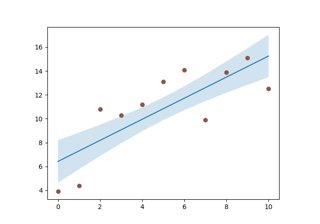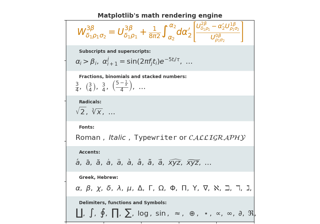# matplotlib.pyplot.fill_between¶

matplotlib.pyplot.fill_between(x, y1, y2=0, where=None, interpolate=False, step=None, *, data=None, **kwargs)[source]

Fill the area between two horizontal curves.

The curves are defined by the points (x, y1) and (x, y2). This creates one or multiple polygons describing the filled area.

You may exclude some horizontal sections from filling using where.

By default, the edges connect the given points directly. Use step if the filling should be a step function, i.e. constant in between x.

Parameters:
xarray (length N)

The x coordinates of the nodes defining the curves.

y1array (length N) or scalar

The y coordinates of the nodes defining the first curve.

y2array (length N) or scalar, default: 0

The y coordinates of the nodes defining the second curve.

wherearray of bool (length N), optional

Define where to exclude some horizontal regions from being filled. The filled regions are defined by the coordinates x[where]. More precisely, fill between x[i] and x[i+1] if where[i] and where[i+1]. Note that this definition implies that an isolated True value between two False values in where will not result in filling. Both sides of the True position remain unfilled due to the adjacent False values.

interpolatebool, default: False

This option is only relevant if where is used and the two curves are crossing each other.

Semantically, where is often used for y1 > y2 or similar. By default, the nodes of the polygon defining the filled region will only be placed at the positions in the x array. Such a polygon cannot describe the above semantics close to the intersection. The x-sections containing the intersection are simply clipped.

Setting interpolate to True will calculate the actual intersection point and extend the filled region up to this point.

step{'pre', 'post', 'mid'}, optional

Define step if the filling should be a step function, i.e. constant in between x. The value determines where the step will occur:

• 'pre': The y value is continued constantly to the left from every x position, i.e. the interval (x[i-1], x[i]] has the value y[i].
• 'post': The y value is continued constantly to the right from every x position, i.e. the interval [x[i], x[i+1]) has the value y[i].
• 'mid': Steps occur half-way between the x positions.
Returns:
PolyCollection

A PolyCollection containing the plotted polygons.

Other Parameters:
**kwargs

All other keyword arguments are passed on to PolyCollection. They control the Polygon properties:

Property Description
agg_filter a filter function, which takes a (m, n, 3) float array and a dpi value, and returns a (m, n, 3) array
alpha array-like or scalar or None
animated bool
antialiased or aa or antialiaseds bool or list of bools
array ndarray or None
capstyle CapStyle or {'butt', 'projecting', 'round'}
clim (vmin: float, vmax: float)
clip_box Bbox
clip_on bool
clip_path Patch or (Path, Transform) or None
cmap Colormap or str or None
color color or list of rgba tuples
contains unknown
edgecolor or ec or edgecolors color or list of colors or 'face'
facecolor or facecolors or fc color or list of colors
figure Figure
gid str
hatch {'/', '\', '|', '-', '+', 'x', 'o', 'O', '.', '*'}
in_layout bool
joinstyle JoinStyle or {'miter', 'round', 'bevel'}
label object
linestyle or dashes or linestyles or ls str or tuple or list thereof
linewidth or linewidths or lw float or list of floats
norm Normalize or None
offset_position unknown
offsets (N, 2) or (2,) array-like
path_effects AbstractPathEffect
picker None or bool or float or callable
pickradius float
rasterized bool
sketch_params (scale: float, length: float, randomness: float)
snap bool or None
transform Transform
url str
urls list of str or None
visible bool
zorder float

fill_between
Fill between two sets of y-values.
fill_betweenx
Fill between two sets of x-values.

Notes

Note

In addition to the above described arguments, this function can take a data keyword argument. If such a data argument is given, the following arguments can also be string s, which is interpreted as data[s] (unless this raises an exception): x, y1, y2, where.

Objects passed as data must support item access (data[s]) and membership test (s in data).

## Examples using matplotlib.pyplot.fill_between¶